## AML Template Tutorial

### Avatar Idle

 Lets face it, no matter how we hard try, we just cant stay completely still unless we are kung fu masters meditating. People shift their weight, adjust their ties, scratch their heads, look around and check the time on their watches. In this lesson, you will learn how to add multiple idle motions as well as learn how to set the occurrences so your characters will be more realistic. Add idle motions one by one and define motion clips for each idle command. When you have more than one idle command the most important setting for you to define "OccurTimes". Set the appear ratio for each idle then when plays they appear randomly from the ratio you define in OccurTimes. Select Idle Command from the command drop down list. Click "Add".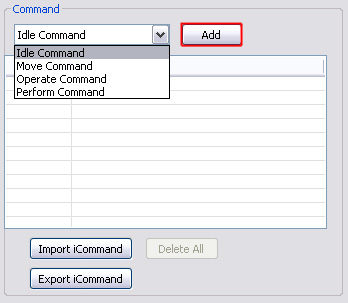Rename the command name to "Idle_01".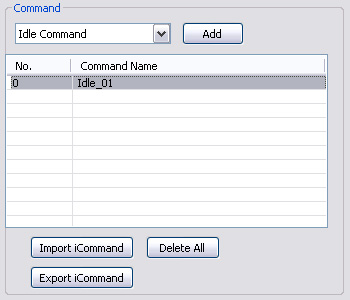Set the OccurTimes for the selected idle command to "15". How are the OccurTimes calculated? 100 * (Command OccurTimes) / (Total OccurTimes) = (Idle Command Appearance Ratio) %. E.g. When AML has two idle commands and the first OccurTimes set to "10" and the second OccurTimes set to "40". Then the first commands appearance rate will be "100 * 10 / ( 10 + 40 ) = 20%".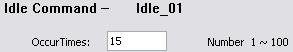100 * 15 / ( 15 + 65 + 100) = 8% Go to ClipSeq section, add an Animation Clip.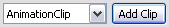Load an idle motion from the default motion folder, C:\Program Files\Reallusion\iClone 3\Template\iClone Template\Motion\iMotion_Trey\Idle.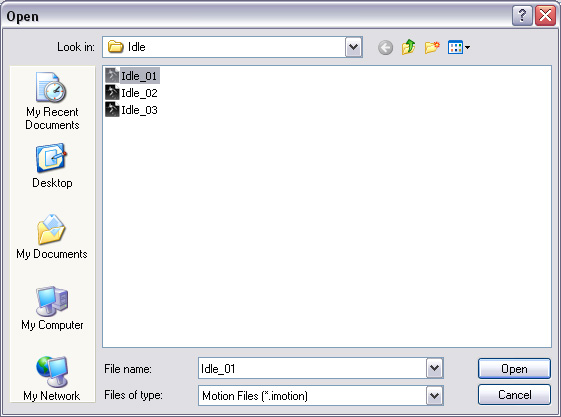Rename the animation clip name to "Default_M_idle_00".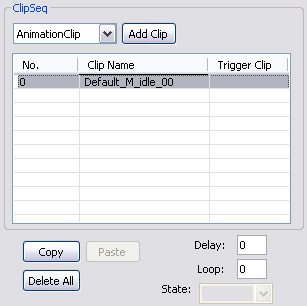Set the number of loops for the animation clip in selection. 0 represents infinite and 1 and up represents the number of times the clip loops. This loops the motion as many times as you defined.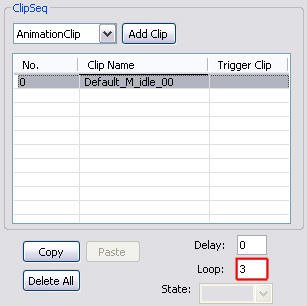Leave the Animation Clip's attributes as default. Add one more Idle Command and rename it "Idle_02".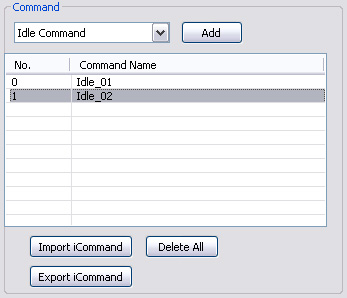Set OccurTimes to 65. How are the OccurTimes calculated? 100 * (Command OccurTimes) / (Total OccurTimes) % = (Idle Command Appearance Ratio). E.g. When AML has two idle commands and the first OccurTimes set to "10" and the second OccurTimes set to "40". Then the first commands appearance rate will be "100 * 10 / ( 10 + 40 ) = 20%".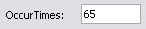100 * 65 / ( 15 + 65 + 100) = 36% Add a new Animation Clip and browse to "Idle_02" in the Trey's idle motion folder.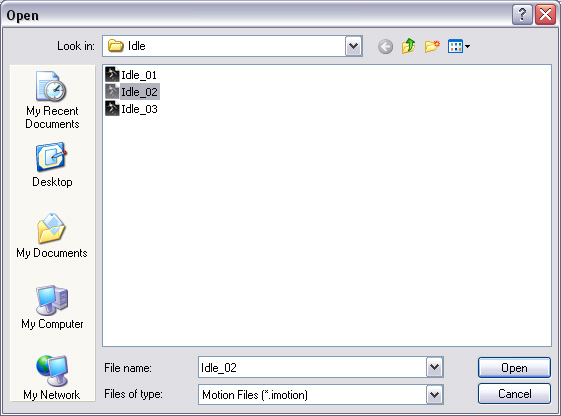Rename the clip to "Default_M_idle_01". Set number of loops to "1".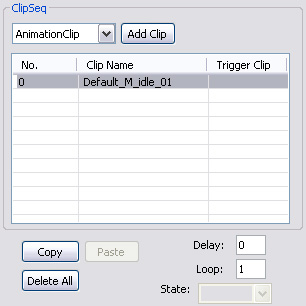Follow the steps above and add a third idle motion. Set the occur times to 100.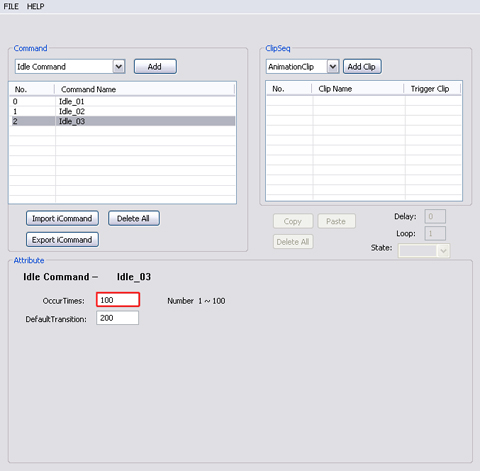100 * 100 / ( 15 + 65 + 100) = 56% Add an animation clip and rename it to "Default_M_idle_02". Set number of loops.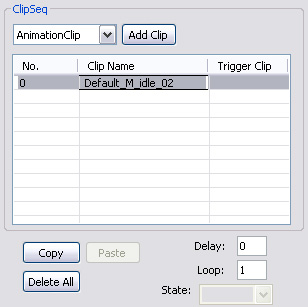Leave the clip's attribute as default and make sure the clip location is correct.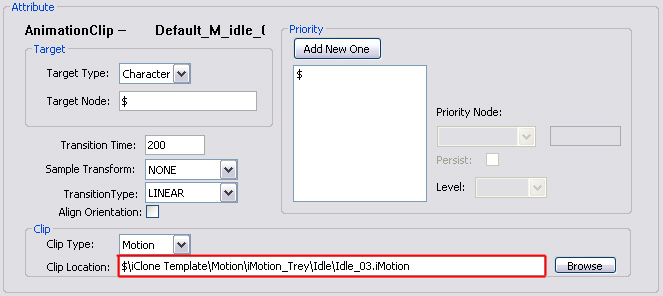Save the script and import into the characters in iClone3.